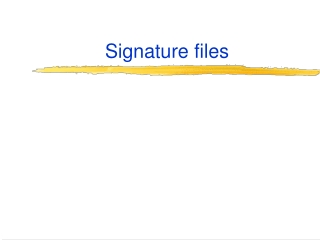DownloadDownload PresentationSignature files

Signature files

Télécharger la présentationSignature files

- - - - - - - - - - - - - - - - - - - - - - - - - - - E N D - - - - - - - - - - - - - - - - - - - - - - - - - - -
Presentation Transcript

1. Signature files

2. Signature Files • Important alternative to inverted indexes. • Given a document, the signature is calculated as follows. • First, each word (term) is hashed into a bit-vector. • Then, these bit-vectors, are OR-ed to form that document’s signature. • Three main issues related to using signature files: 1. Generating signatures 2. Boolean logic on signatures 3. Accessing signatures

3. Signatures

4. Computing Signatures • W: width of signatures (in the range of 1,000 to 10,000). • Each term (word) sets b bits out of these W bits. • To generate the hash string of a term: for i = 1 to b sig[ hi(term) % W ] = 1 • Each hi() is a hash function. • The signature of the document is generated by OR-ing the hash strings of all its terms.

5. Example • Sometimes some hash strings end up with less then b bits being set. Why? • Because a term may get hashed to the same location by two hash functions.

6. Query logic on signature files • Fact 1. A document contains term Tonly if all the bits that are set by T’s hash string are also set in the document’s signature. • Fact 2. However, a document’s signature that has all these bits set doesn’t necessarily mean that T appears in that document. Why? • Because the particular “1”-bits can be set by some other terms.

7. Query logic on signature files Query All bits set Some bits missing T Maybe No Not T Maybe Yes

8. Three Valued Logic

9. Search efficiency How to search for a set of given terms? • Naïve way: Access the signatures of all the documents. • For each document, the signature is compared with the OR-ed hash string of the query • to see whether for each “1”-bit of that hash string, the descriptor has its corresponding bits set. • This implies reading the entire signature set! • Not affordable in practice. • Better: Use bitslices.

10. Bitslices • Signature files have to be stored on disk in transposed form. • Example: Search for “cold.” • Retrieve the bitslices for “cold” and then AND them.

11. What should be the signature width? W = width of the signature (we are trying to determine best) b = bit slices per query (equals number of accesses, we specify what we tolerate) z = expected number of false match documents (we specify what we tolerate) f = number of (term, document) pairs N = number of documents B = average of "on"-bits per document. B = b * (f/N) p = probability that a random bit in a document signature is "on" p = 1- [(W-1)/W]B Probability for a bit to remain "off" is: [(W-1)/W]B since it must avoid selection B times, and the probability of not being selected once is (W-1)/W. z= expected number of false matches z=pb*N A false match document (FMD) requires that the bit slices of the query agree on the "on"-bit for the FMD. So, the probability for a random document to be a false match is pb (see note). The expected number of FMDs is z=pb*N.

12. Random document – note • “Probability” of a good document to be a match is of course “1” (that’s a certain event). • Probability for a false match is the probability for a random document to end up being a match in the index (pb).

13. What should be the signature width? W = width of the signature (we are trying to determine best) b = bit slices per query (equals number of accesses, we specify what we tolerate) z = expected number of false match documents (we specify what we tolerate) f = number of (term, document) pairs N = number of documents B = average of "on"-bits per document. p = probability that a random bit in a document signature is "on" B = b * (f/N) (1) p = 1-[(W-1)/W]B (2) z=pb*N (3) We can derive W from (2): W = 1/[1-(1-p)1/B] and substitute B using (1) and p using (3).

14. TREC Collection example W = width of the signature (we are trying to determine best) b = bit slices per query (equals number of accesses, we specify what we tolerate) z = expected number of false match documents (we specify what we tolerate) f = number of (term, document) pairs N = number of documents B = average of "on"-bits per document. p = probability that a random bit in a document signature is "on" b = 8, z=1, N=741,856, f=135,017,792 We derive: p=0.185 f/N = 182 unique terms for the average document. B = 1,456 So, W = 7,134 This collection of 741,856 documents would need: 7,134 * 741,856 bits, that is 7,134 * 741,856 / 8 = 661,550,088 bytes 631Mb.